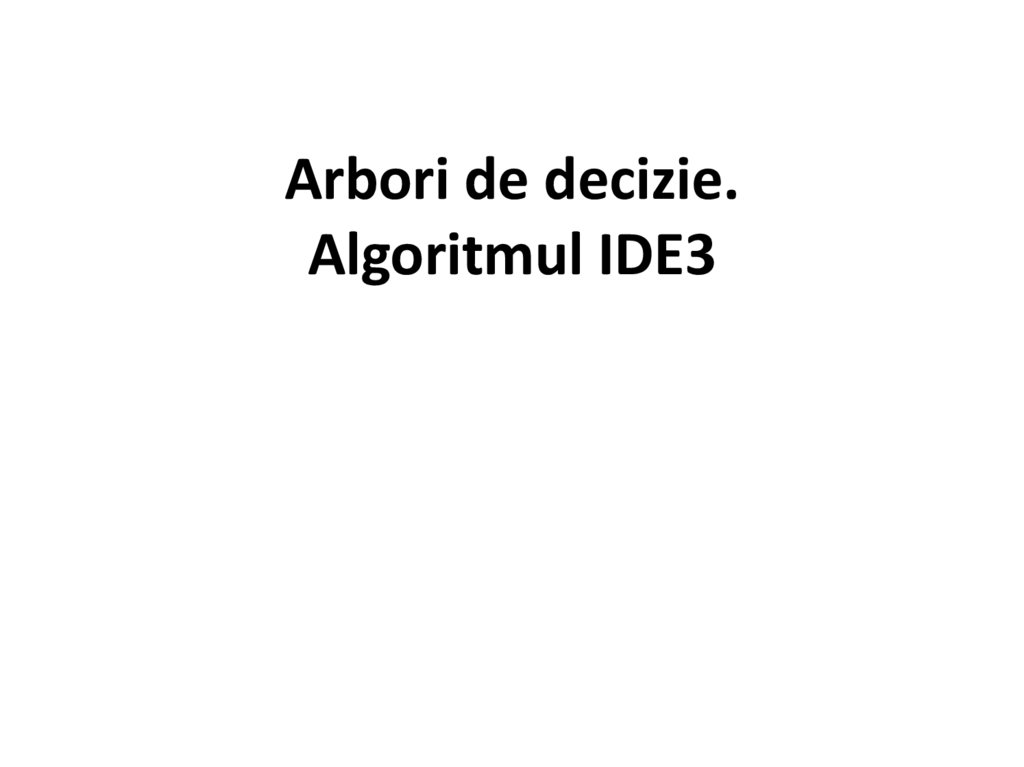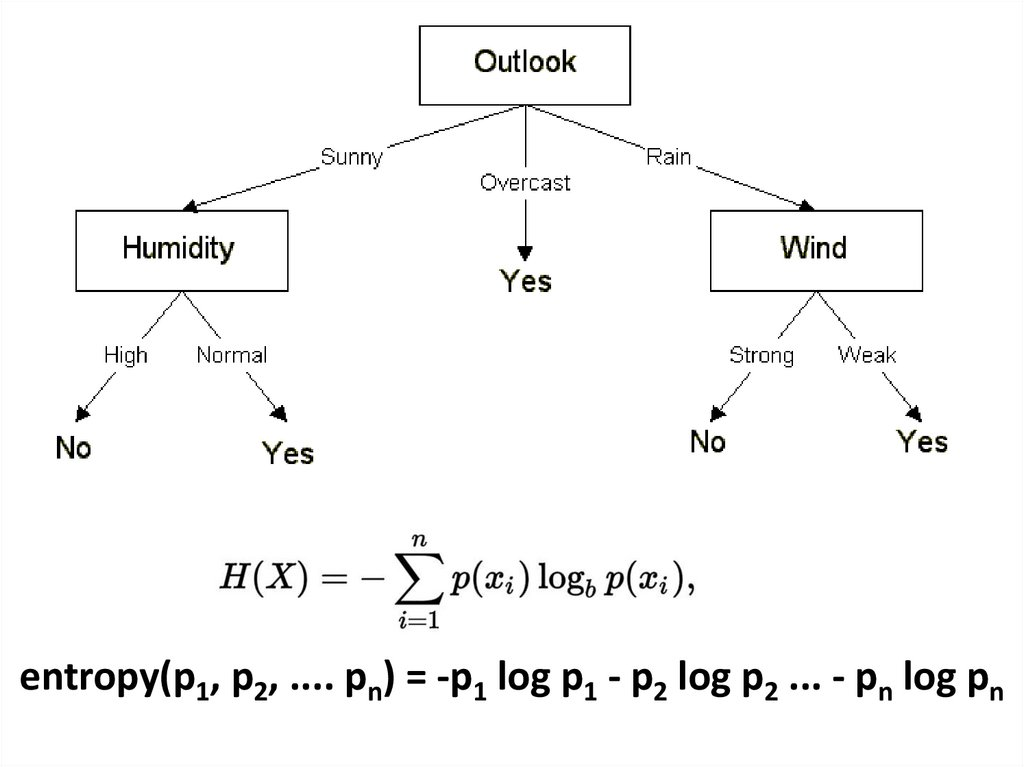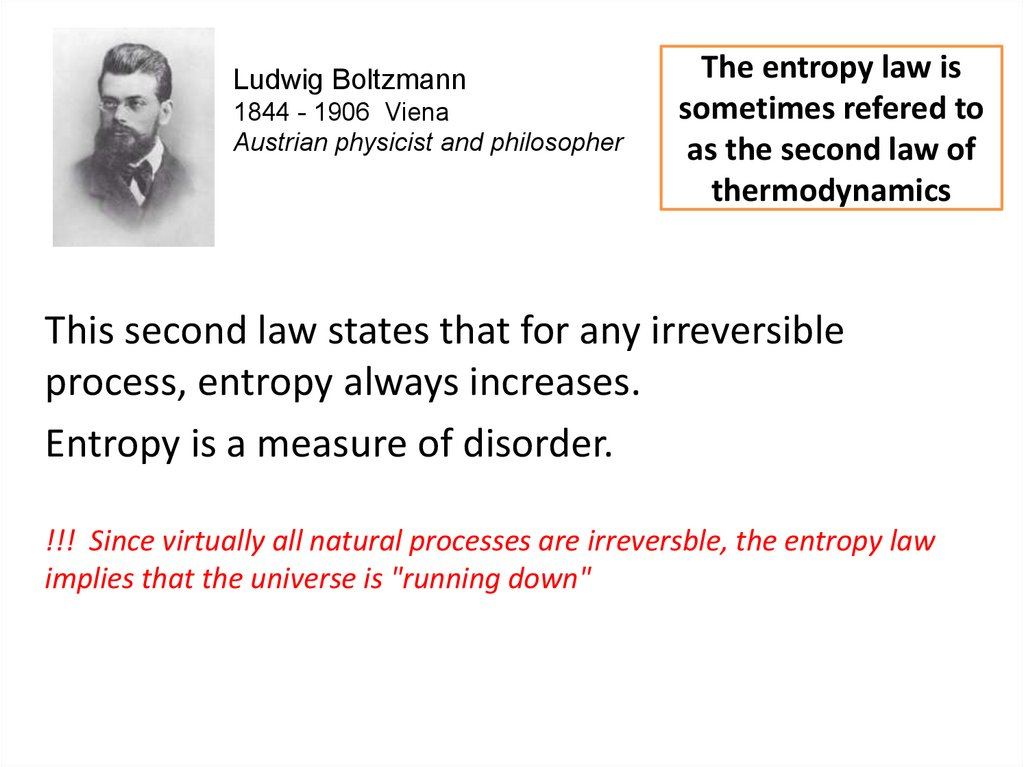# Arbori de decizie. Algoritmul IDE3

## 1.

Arbori de decizie.
Algoritmul IDE3

## 2.

entropy(p1, p2, .... pn) = -p1 log p1 - p2 log p2 ... - pn log pn

## 3.

Ludwig Boltzmann
1844 - 1906 Viena
Austrian physicist and philosopher
The entropy law is
sometimes refered to
as the second law of
thermodynamics
This second law states that for any irreversible
process, entropy always increases.
Entropy is a measure of disorder.
!!! Since virtually all natural processes are irreversble, the entropy law
implies that the universe is "running down"

## 5.

Entropy can be seen as a measure of the quality
of energy:
Low entropy sources of energy are of high
quality. Such energy sources have a high energy
density.
High entropy sources of energy are closer to
randomness and are therefore less available for
use

## 6.

Entropy in Information Theory
Claude Shannon
1916 –2001
American mathematician, electrical engineer,
and cryptographer known as "the father
of information theory".
- Claude Shannon transferred some of these
ideas to the world of information
processing. Information is associated by him
with low entropy.
- Contrasted with information is "noise",
randomness, high entropy.

## 7.

• At the extreme of no information are
random number.
• Of course, data may only look random.
But there may be hidden patterns, information
in the data. The whole point of ML is to dig out
the patterns. The descovered patterns are
usually presented as rules or decision trees.
Shannon's information theory can be used to
construct decision trees.

## 8.

A collection of random numbers has maximum entropy

## 9.

Entropy in Information Theory
- Shannon defined the entropy Η (Greek
capital letter eta) of a discrete variable X with
possible values {x1, ..., xn} and probability
p as:
- where b is 2 in case when information
measure is the bit.

## 10.

Example
• Presupunem evenimentul aruncării unui zar
cu 6 fețe. Valorile variabilei X sunt {1,2,3,4,5,6}
iar probabilitățile obținerii oricărei valori sunt
egale.
- În acest caz entropia este:
bits.

## 13.

There are 14 instances: 9 yes, 5 no
TotalEntropy([9,5]) = 0.940 bits.
Entropyoutlook([2,3], [4,0],[3,2]) = (5/14) * 0.971 + (4/14) * 0.0 + (5/14) * 0.971 = 0.693bits
InfoGain = 0.940 – 0.693 = 0.247 bits of information

## 14.

gain(outlook) = 0.940 - 0.693 = 0.247 bits of information.
gain(temperature) = 0.029 bits
gain(humidity) = 0.152 bits
gain(windy) = 0.048 bits

Thank you